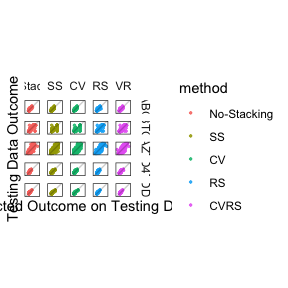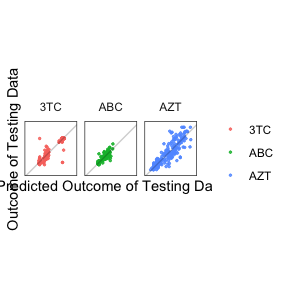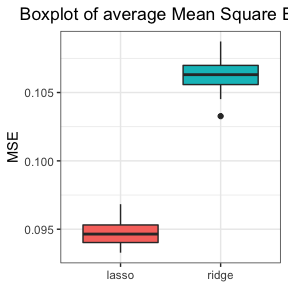# Introduction

Stacking is an ensemble machine learning algorithm, which allows multiple prediction tasks to borrow information from each other. The Standard Stacking (SS) for multiple outcomes prediction problem consists of two steps of model fitting. In step 1, the individual models are fitted to predict resistance of each drug separately. In step 2, the combiner models are fitted to integrate information from predictions of individual models and to make final predictions. Two variations of stacking algorithm are proposed. They are Cross-Validation Stacking (CVS) and Residual Stacking (RS).

The motivation for Cross-Validation Stacking (CVS) is that the combiner models are learned from the Step one ‘fitted’ values $$\hat Y$$ in SS, and the final predictions are calculated from the step one ‘predicted’ values using new data. The two types of quantities are different, for instance, in linear regression models, it is known that predicted values have larger variance than fitted values. In CVS, The ‘fitted’ values $$\hat Y$$ in SS step 1 is replaced the cross-validation predicted values $$\hat Y ^ *$$ to deal with the discrepancy between fitted values and predicted values.

Sometimes in SS, the step 1 prediction accuracy may be compromised by stacking when step 1 already provides accurate predictions. To ameliorate this problem, Residual Stacking (RS) is proposed. In RS, all information learned from step 1 models is retained. In step 2, the outcome is learned using all other fitted value exclude the outcome as the predictors to predict the residual of the outcome. Then, the predictions in step 1 will be revised using the residuals.

The MTPS package is a flexible package which implements the Revised Stacking algorithms. It can fit models and make predictions using the above two variations of stacking algorithms and their combination. It provides various base learners to train models in step 1 and step 2. Users can modify default parameters in base learners provided in the package. It is also available for users to create new base learners and use those learners to fit models. This package can fit models on continuous, binary and mix outcomes. An interface for model evaluation using cross-validation method is available in the package.

# Example Data

As with any package, you first need to load it with the following command

library(MTPS)

The HIV Drug Resistance Database is used as the example data in the package. In the HIV database, the resistance of five Nucleoside RT Inhibitor (NRTI) drugs were used as multivariate outcomes, including Lamivudine (3TC), Abacavir(ABC), Zidovudine (AZT), Stavudine (D4T), Didanosine (DDI). The mutation variables are used as the predictors. Some mutation variables were removed as they do not contain enough variation. The final outcome data is a matrix of size 1246 × 5, and the predictor data is a matrix of 1246 × 228 values, which is provided in the package called “HIV”. In the example data in the package, “YY” refers the outcome data and “XX” refers the predictor data.

data("HIV")
head(YY)
##          ABC       3TC         AZT        D4T         DDI
## 1 0.63346846 2.3010300  0.59106461 0.14612804  0.07918125
## 3 0.04139269 0.1461280  1.44715803 0.00000000 -0.09691001
## 4 0.17609126 0.2552725  0.85125835 0.07918125  0.04139269
## 6 0.85125835 2.3010300 -0.09691001 0.11394335  0.27875360
## 7 0.71600334 0.4065402  0.82607480 0.73239376  0.72427587
## 8 0.77085201 2.3010300  0.27875360 0.11394335  0.23044892
XX[8:9, 7:10]
##    X.11K X.11R X.20K X.20R
## 10     1     1     0     0
## 11     0     0     0     1
dim(YY)
##  1246    5
dim(XX)
##  1246  228

# Model Fittig and Prediction

## Revised Stacking Algorithm for Continuous Outcome

The HIV data set is used as the example. To illustrate how to fit the model and make predictions, we first split the HIV data into 2 parts, the training data and testing data.

set.seed(12345)
xmat <- as.matrix(XX)
ymat <- as.matrix(YY)
nobs <- nrow(xmat)
id <- createFolds(rowMeans(XX), k=5, list=F)
training.id <- sample(seq_len(nobs), size = 0.8 * nobs)
y.train <- ymat[training.id, ]
y.test  <- ymat[-training.id, ]
x.train <- xmat[training.id, ]
x.test  <- xmat[-training.id, ]

The r multiFit function fits individual models for each outcome separately, referring the non-stacking algorithm. The following code fits generalized linear models with elastic net regularization on each outcome.

# no stacking
fit.mult <- multiFit(xmat = x.train, ymat = y.train, method = glmnet.ridge, family = "gaussian")

To set up the stacking algorithm, A list of algorithms for step one and step two need to be specified. For continuous outcome we need to specify r family = "gaussian". We also use r cv and r residual argument to specify whether we want to use Cross-Validation Stacking (CVS) or Residual Stacking (RS) or their combination. The default value for r cv is “FALSE” and for r residual is “TRUE”, referring to the residual stacking algorithm. The following code fits models using Standard Stacking algorithm (SS), Cross-Validation Stacking (CVS), Residual Stacking (RS) and Cross-Validation Residual Stacking (CVRS) with r MTPS function.

In the example, generalized linear models with elastic net regularization are used to fit models in the step one and tree models are applied in the step two for each outcome.

# Standard Stacking
fit.ss <- MTPS(xmat = x.train, ymat = y.train, family = "gaussian",
cv = FALSE, residual = FALSE,
method.step1 = glmnet.ridge,
method.step2 = rpart1)
# Cross-Validation Stacking
fit.cv <- MTPS(xmat = x.train, ymat = y.train, family = "gaussian",
cv = TRUE, residual = FALSE,
method.step1 = glmnet.ridge,
method.step2 = rpart1)
# Residual Stacking
fit.rs <- MTPS(xmat = x.train, ymat = y.train, family = "gaussian",
cv = FALSE, residual = TRUE,
method.step1 = glmnet.ridge,
method.step2 = rpart1)
# Cross-Validation Residual Stacking
fit.cvrs <- MTPS(xmat = x.train, ymat = y.train, family = "gaussian",
cv = TRUE, residual = TRUE,
method.step1 = glmnet.ridge,
method.step2 = rpart1)

The r predict function returns the predicted value for the outcome on the testing data.

# no stacking
pred.mult <- predict(fit.mult, x.test)
# Standard Stacking
pred.ss <- predict(fit.ss, x.test)
# Cross-Validation Stacking
pred.cv <- predict(fit.cv, x.test)
# Residual Stacking
pred.rs <- predict(fit.rs, x.test)
# Cross-Validation Residual Stacking
pred.cvrs <- predict(fit.cvrs, x.test)

To visualize the above prediction, we plot the predicted value versus the actual outcomes of testing data for above algorithms.

library(ggplot2)
library(reshape2)
n.test <- nrow(x.test)
ctn.plot.data_matrix <- cbind(rbind(pred.mult, pred.ss, pred.cv, pred.rs, pred.cvrs), y.test[rep(seq_len(n.test), 5), ])
ctn.plot.data <-data.frame(rep(c("No-Stacking", "SS", "CV", "RS", "CVRS"), each = n.test),ctn.plot.data_matrix)
colnames(ctn.plot.data) <- c("method", paste0(rep("pred.", ncol(y.test)), colnames(y.test)), colnames(y.test))
dm1 <- melt(ctn.plot.data[,c("method","ABC","3TC","AZT","D4T", "DDI")], id=c("method"))
dm2 <- melt(ctn.plot.data[,c("method","pred.ABC","pred.3TC","pred.AZT","pred.D4T", "pred.DDI")], id=c("method"))
ctn.plot.data <- cbind(dm1, dm2[, -1])
colnames(ctn.plot.data) <- c("method", "Y", "yVal", "predict", "predictVal")
ctn.plot.data$method <- factor(ctn.plot.data$method, unique(as.character(ctn.plot.data$method))) ctn.plot.data$yVal <- as.numeric(ctn.plot.data$yVal) ctn.plot.data$predictVal <- as.numeric(ctn.plot.data$predictVal) ggplot(ctn.plot.data) + geom_point(aes(x=predictVal, y=yVal, color=method), size = 0.5, alpha = 0.8) + geom_abline(slope=1, alpha = 0.2) + coord_fixed() + ylab("Testing Data Outcome") + xlab("Predicted Outcome on Testing Data") + scale_x_discrete(breaks = NULL) + scale_y_discrete(breaks = NULL) + theme_bw() + theme(axis.text = element_blank(), strip.placement = "outside", strip.background = element_blank()) + facet_grid(Y~method)## Revised Stacking Algorithm for Binary Outcome The MTPS package can fit models and make predictions when the outcome is binary. We use the HIV data to illustrate the usage. First we need convert the continuous outcome in the HIV data into binary outcome. the HIV database website recommended cutoff values to convert IC50 ratios to binary outcomes are 3TC = 3, ABC = 2, AZT = 3, D4T = 1.5, and DDI = 1.5. Then, using similar approach the data is split into training data and testing data. # https://hivdb.stanford.edu/pages/published_analysis/genophenoPNAS2006/CUTOFFS/drug.cutoffs # cutoff value to be used to define drug resistent cutoffs <- c(2,3,3,1.5,1.5) ymat.bin <- ymat xmat.bin <- xmat for(ii in 1:5) ymat.bin[,ii] <- (10^ymat[,ii] < cutoffs[ii]) * 1 y.train.bin <- ymat.bin[training.id, ] y.test.bin <- ymat.bin[-training.id, ] x.train.bin <- xmat.bin[training.id, ] x.test.bin <- xmat.bin[-training.id, ] For binary outcomes, the residual type can be deviance',pearson’ or raw' for Deviance residual, Pearson residual or raw residual, respectively. The default value for the r residualis "deviance". The r resid.std argument determines whether or not to standardized the residuals and the default value is “FALSE”. Note that for Residual Stacking algorithm, the step 2 method must be able to fit continuous outcomes. Though we are making predictions on binary data, the step 2 in residual stacking is to make predictions on the residuals Therefore, we need use a method that can fit models for continuous outcomes. The following code fit models using the Residual Stacking algorithms with standardized Pearson residual. The step one uses tree method and step two uses linear regression method. fit.prs.std <- MTPS(xmat = x.train.bin, ymat=y.train.bin, family = "binomial", cv = FALSE, residual = TRUE, method.step1 = rpart1, method.step2 = lm1, resid.type = "pearson", resid.std = TRUE) pred.prs.std <- predict(fit.prs.std, x.test.bin) To see how the model performs we print the confusion matrices of each outcome on the testing data. 0.5 is used as the threshold for the prediction. for (yy in 1 : ncol(y.test.bin)) { print(colnames(y.test.bin)[yy]) print(table((pred.prs.std[,yy] > 0.5) * 1, y.test.bin[,yy])) } ##  "ABC" ## ## 0 1 ## 0 139 5 ## 1 10 96 ##  "3TC" ## ## 0 1 ## 0 131 8 ## 1 10 101 ##  "AZT" ## ## 0 1 ## 0 110 15 ## 1 2 123 ##  "D4T" ## ## 0 1 ## 0 99 17 ## 1 9 125 ##  "DDI" ## ## 0 1 ## 0 114 26 ## 1 6 104 ## Revised Stacking Algorithm for Mix Outcome For illustration purpose, the first three columns of the outcome in the HIV data and last two columns of the binary outcome HIV data are combined for the mix outcome example. ymat.mix <- cbind(ymat[,1:3], ymat.bin[,4:5]) xmat.mix <- xmat y.train.mix <- ymat.mix[training.id, ] y.test.mix <- ymat.mix[-training.id, ] x.train.mix <- xmat.mix[training.id, ] x.test.mix <- xmat.mix[-training.id, ] When the outcome variable is a combination of continuous and binary outcomes, it is necessary to specify each outcome type in the r family argument. For example, we fit the model using Residual Stacking algorithm. The first step uses LASSO and the second step uses tree method. fit.mix.rs <- MTPS(xmat = x.train.mix, ymat = y.train.mix, family=c("gaussian","gaussian","gaussian","binomial","binomial"), cv = FALSE, residual = TRUE, method.step1 = glmnet.lasso, method.step2 = rpart1) pred.mix.rs <- predict(fit.mix.rs, x.test.mix) The following code draw the scatter plot of predicted value versus the actual outcomes on the first three columns of testing data, and the two confusion matrices for the last two columns of the testing data using 0.5 as the cutoff value. n.test <- nrow(x.test) mix.plot.data <- cbind(rep(colnames(y.test.mix)[1:3], each=nrow(y.test.mix)), rbind(cbind(pred.mix.rs[, 1], y.test.mix[, 1]), cbind(pred.mix.rs[, 2], y.test.mix[, 2]), cbind(pred.mix.rs[, 3], y.test.mix[, 3]))) colnames(mix.plot.data) <- c("Y", "predict", "outcome") mix.plot.data <- as.data.frame(mix.plot.data) mix.plot.data$predict <- as.numeric(as.character(mix.plot.data$predict)) mix.plot.data$outcome <- as.numeric(as.character(mix.plot.data$outcome)) ggplot(mix.plot.data) + geom_point(aes(x=predict, y=outcome, color=Y), size = 0.5, alpha = 0.8) + ylab("Outcome of Testing Data") + xlab("Predicted Outcome of Testing Data") + scale_x_discrete(breaks = NULL) + scale_y_discrete(breaks = NULL) + geom_abline(slope=1, alpha = 0.2) + coord_fixed() + theme_bw() + theme(legend.title = element_blank(), axis.text = element_blank(), strip.placement = "outside", strip.background = element_blank()) + facet_grid(~Y)for (yy in 4 : 5) { print(colnames(y.test.mix)[yy]) print(table((pred.mix.rs[,yy] > 0.5) * 1, y.test.mix[,yy])) } ##  "D4T" ## ## 0 1 ## 0 100 18 ## 1 8 124 ##  "DDI" ## ## 0 1 ## 0 113 20 ## 1 7 110 # Specifying Models in Step 1 and Step 2 ## Specify Different Base Learners for Different Outcomes within One Step In the above examples, only one base learner is used in each step. The MTPS package allow users to choose different base learners within one step for each outcome according to the outcome properties. The following example use different base learners for different outcomes. In step one, the first three outcomes are fitted by the LASSO model and the last two outcomes are fitted by the linear regression methods. In the second step, the first outcome is fitted by the tree methods and the last four outcome is fitted by the LASSO model. By default the residual stacking algorithm is applied. fit.mixOut <- MTPS(xmat=x.train, ymat=y.train, family="gaussian", method.step1 = c(glmnet.lasso,glmnet.lasso,glmnet.lasso,lm1,lm1), method.step2 = c(rpart1,glmnet.lasso,glmnet.lasso,glmnet.lasso,glmnet.lasso)) pred <- predict(fit.mixOut, x.test) ## User-Defined Base Learners Apart from giving base learners in the package, users are able to define new methods to fit models in step one or step two. Users can either change default parameters in our provided base learners or define their new methods. ### Modify Arguments for Existing Base Learners The MTPS package provides several methods to fit models. Some of these methods has some default arguments. For example, the glmnet1 method fit a generalized linear model with elasticnet regularization. By default, using cross validation, the model chose the best alpha in 0, 0.2, 0.4, 0.6, 0.8 and 1. If user want to fit a LASSO or ridge model, that is, fix the value of alpha equals 1 or 0. (glmnet.lasso and glmnet.ridge method are available in the MTPS package) users can use the r modify.parameter function to pass arguments. glmnet.lasso <- modify.parameter (glmnet1, alpha=1) glmnet.ridge <- modify.parameter (glmnet1, alpha=0) ### Define New Base Learners Apart from modifying model parameters, users can define new methods. In order to use the new methods, users need to make sure that the input and output format is consistent with existing methods. The new method should be defined as a function with predictor xmat (matrix), outcome ymat (matrix with 1 column or a vector), and the outcome family as inputs. The outputs of the function should be a list which contains three elements called “model”, “y.fitted” and “predFun”. The “model” element is the method object (which will be used in the corresponding predict function). The “y.fitted” element should be a matrix / dataframe of fitted value and the “predFun” should be a r predict function for the method object. The following example shows how to define a method. glm1 <- function(xmat, ymat, family, ...) { tmp0 <- data.frame(yy=ymat, xmat) model <- glm(yy~., data=tmp0, family=family, ...) y.fitted <- fitted(model) predFun <- function(model,xnew){ predict(model, newdata=data.frame(xnew), type="response") } return(list(model=model,y.fitted=y.fitted, predFun=predFun)) } AAfter defining new base learners, Users can pass these methods in method arguments in the MTPS function. # Performance Comparison Cross-Validation is a popular way to compare different methods. Here the cross-validation method is used as an example to evaluate the performance of choice of base learners in the stacking algorithms. The r cv.MTPS function provide a simple interface for model evaluation using cross-validation. It returns the mean square error for continuous outcomes and AUC, accuracy, recall and precision for binary outcomes from cross-validation predictions. Following is an example to use 5-fold Cross-Validation method to compare using LASSO and ridge models in step 1 of residual stacking. Both of them are followed by linear regression in step 2. The cross-validation process can be repeated to compare the these two methods. For speed reasons here we only repeat the above process for 20 times and plot the boxplot of their MSE. nsim <- 20 mse.lasso.lm <- matrix(NA, nrow = nsim, ncol = ncol(ymat)) mse.lasso.lm <- as.data.frame(mse.lasso.lm) colnames(mse.lasso.lm) <-colnames(ymat) mse.ridge.lm <- mse.lasso.lm for (ii in 1:nsim) { set.seed(ii) # lasso stacking with lm mse.lasso.lm[ii,] <- cv.MTPS(xmat, ymat, family="gaussian", cv = FALSE, residual = TRUE, method.step1=glmnet.lasso, method.step2=lm1, resid.std=FALSE)$continuous
# ridge stacking with lm
mse.ridge.lm[ii,] <- cv.MTPS(xmat, ymat, family="gaussian",
cv = FALSE, residual = TRUE,
method.step1=glmnet.ridge, method.step2=lm1,
resid.std=FALSE)\$continuous
}
# box plot
mse.data <- data.frame(lasso=apply(mse.lasso.lm,1,mean),
ridge=apply(mse.ridge.lm,1,mean))
mse.data <- melt(mse.data,measure.vars = c("lasso","ridge"))

colnames(mse.data) <- c("Learner", "MSE")
ggplot(mse.data) +
geom_boxplot(aes(x=Learner, y=MSE, fill=Learner)) +
ggtitle("Boxplot of average Mean Square Error") +
theme_bw() +
theme(legend.position = "none",
plot.title = element_text(hjust = 0.5),
axis.title.x = element_blank())`Similarly, the above cross-validation process can be applied when the outcome is binary or a combination of continuous and binary outcomes. Some possible evaluation methods are accuracy rate, area under curve (AUC), etc.

# Reference

Dimitriadou, E., Hornik, K., Leisch, F., Meyer, D., and Weingessel, A. (2008).
Misc functions of the Department of Statistics (e1071), TU Wien.
R package,1,5-24.

Friedman, J., Hastie, T., and Tibshirani, R. (2010).
Regularization Paths for Generalized Linear Models via Coordinate Descent
Journal of Statistical Software, Vol. 33(1) 1-22.

Kuhn, M. (2008).
Building Predictive Models in R Using the caret Package.
Journal of Statistical Software, Vol. 28(5), 1-26.

Ripley, B.D. (1996).
Pattern Recognition and neural networks
Cambridge University Press.

Venables, W. N., & Ripley, B. D. (2013). Modern applied statistics with S-PLUS.Springer Science & Business Media.

Xing, L., Lesperance, M., & Zhang, X. (2019).
Simultaneous prediction of multiple outcomes using revised stacking algorithms
Bioinformatics, https://doi.org/10.1093/bioinformatics/btz531.